The Asentric Factor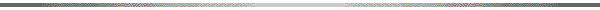It is used in thermodynamic correlations involving fugacity, compressibility factor, enthalpy, and viral coefficients.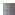Calculate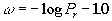Where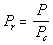Pc is the critical pressure

P is the vapor pressure at Tr = 0.7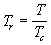Tc is the critical temperature

T is the temperature.Value